# Quiz 10: Valuation of Income Properties: Appraisal and the Market for Capital

Business

Valuation of property Z investment is considering a property for purchase. Company has done extensive market research of the subject property and based on analysis cash flow in term of net operating income in analyzed. a. Net operating income of property is given for year 1 to 8 in the question. Z Corporation wants to own the property for seven year and then sell the property in 8 th year. The growth rate in NOI is 3%, and the Expected return on property is 12%. Value of property today is present value of all the cash flow that property can earn in the future. Terminal value of property is the reversion value at the end of eighth year. Compute the terminal value as follows:Value of property at end of year-7 is \$15,324,111. The current value of property is the present value all the cash inflow in term of net operating income and the terminal value. In order to compute the present value of the all future cash flows use the "NPV" function of spreadsheet. Enter the given values and formulas in the spreadsheet as shown in the image below.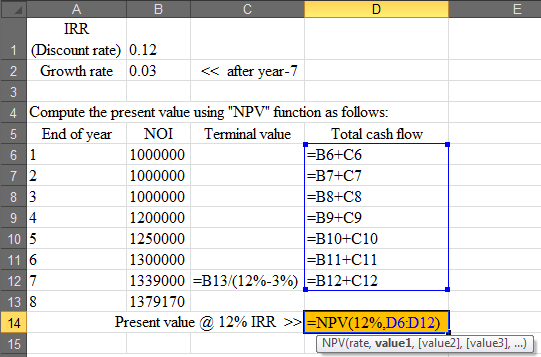Obtained result is provided below.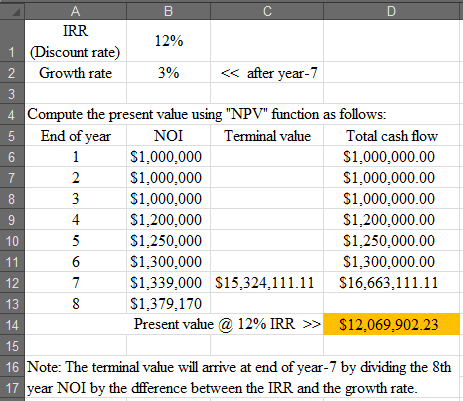Thus, the present value of property is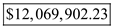. b. Value of RT depends on the value of IRR and the growth rate in NOI. The RT will be the difference between IRR and the growth rate. Compute the terminal capitalization rate (RT) as follows:Thus, value of RT is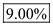. c. Going in Cap rate based on first year NOI is calculated by the first year Net operating income divided by the current value of property. Compute the "going-in" capitalization rate as follows:Thus, going in cap rate based on first year NOI is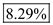d. The terminal capitalization rate is the discount rate used to discount the perpetual cash flow from the property considering the growth rate in the property. It is the difference between the IRR (the required rate of return of investor) and the growth rate in NOI (Net Operating Income) constantly in perpetuity. However, the "going-in" capitalization rate is the percentage return the investor will receive on the value of the property. It is computed by dividing the first year NOI from the property by the present value of the property. The "going-in" capitalization rate is misleading and not useful in comparing properties. This is because it does not consider the NOI of all years from the property.

Appraisal Process: The appraisal procedure is a systematic procedure in which the assessment of estimated market value of a property as on a particular date is built up. The issues related to the work to be done are arranged in this process. The information to be included is procured, ordered, and analyzed. Thereafter various techniques are applied on the analyzed information to get the estimated value. Economic rationale for cost approach: The cost approach says that the individual who is purchasing the property should not pay more than the actual cost of the property in the market. The market price of the property should be equal to the cost paid by the person who is buying the property. The cost of the property should not exceed more than the cost to buy the land and build the structure. Conditions in which cost approach gives best value estimate: The cost approach is most reliable in following reasons that are mentioned below. • New construction of structure does not create problems in estimation of cost. • Old property can have major depreciation which might be difficult to accurately account for estimation of cost. Structures with no prior depreciation or less depreciations are easy for best estimates.

The value of property can be computed here with the help of market valuation approach. This approach considers the expected income from property, growth rate in income, and the required rate of return. It is like finding the present worth of the future cash flows from the property. a. The expected cash flow in next year is \$2,000,000, and it is expected to grow by 4% each year. The discounting rate/required rate of return is 13%. Compute the value of property as follows:Thus, the value of property is. b. The "going-in" cap rates shows the potential of a property to generate the return for the investor. It is generally, computed by dividing the expected annual income by the property value. It can also be computed by subtracting the growth rate in the NOI from the required rate of return. It is useful in comparing properties. Compute the "going-in" cap rates as follows:Thus, the "going-in" cap rate is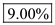. c. The expected cash flow in next year is \$2,000,000, and it is expected to grow by 4% each year. The discounting rate/required rate of return is 12%. Compute the value of property as follows:Thus, the value of property is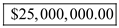. d. The required rate of return indicates the rate of return required by the investors on the comparable properties. The required rate of return and the property value have inverse relationship. The increase in required rate of return will result in the decreased value of property and vice versa is also true. In part a. the required return is 13% and in part c. it is 12%, which indicates 1% decrease in the required rate of return. Here, the increase in value of property is the decrease in required return. Thus, it can be concluded that the decrease in required rate of return is the cause of increase in value of property.